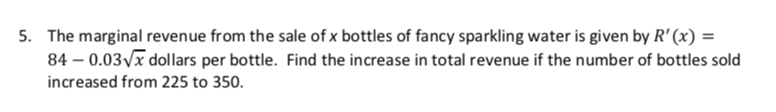# The marginal revenue from the sale of x bottles of fancy sparkling water is given by R' (x)84 0.03 x dollars per bottle. Find the increase in total revenue if the number of bottles soldincreased from 225 to 3505.

Question
2 viewshelp_outlineImage TranscriptioncloseThe marginal revenue from the sale of x bottles of fancy sparkling water is given by R' (x) 84 0.03 x dollars per bottle. Find the increase in total revenue if the number of bottles sold increased from 225 to 350 5. fullscreen
check_circle

Step 1

Given,

Total revenue is the multiplication of price per unit and quantity of goods. After integrating the marginal revenue function with respect to x, total revenue can be find. Which is equal to:

Step 2

If x = 225, total revenue is:

Step 3

Similarly, if x = 350, t...

### Want to see the full answer?

See Solution

#### Want to see this answer and more?

Solutions are written by subject experts who are available 24/7. Questions are typically answered within 1 hour.*

See Solution
*Response times may vary by subject and question.
Tagged in